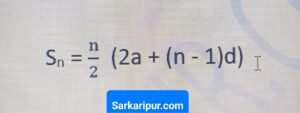# PDF [Chapter 5] – Arithmetic Progressions Class 10 Notes PDF Download

The PDF of Class 10th Chapter 5 Arithmetic Progression Notes PDF has been prepared for the students of class 10th by the Sarkaripur, which will be very important for the preparation of all of you and you are being provided in all the notes PDF.

These notes will be very beneficial for all those students who want to improve their preparation in class 10th and this note will help you a lot to prepare well for your upcoming exams.

The Revision Notes for Class 10th Chapter 5 Arithmetic Progression will help you a lot to improve your preparation and revise your concepts in the right way.

In the revision notes that are being provided to you, along with the notes, examples and formulas have also been given, which will help you a lot in solving the questions that come from Arithmetic Progression and you can study these notes properly for class 10th You can solve all the questions of Chapter 5 Arithmetic Progression.

### IMPORTANT FORMULA OF ARITHMETIC PROGRESSION CLASS 10TH

1. First Term Of AP

> a, a + d, a + 2d, a + 3d, a + 4d, ……., a + (n-1) d

2. Common Difference in Arithmetic Progression

d = a2 – a1 = a3 – a2 = ……. = an – an – 1

3. nth Term of an AP

> an = a + (n – 1) x d

4. Sum of N Term of AP### Solved Example Of Arithematic Progression Class 10th

Example 1 : Find the 10th term of the AP : 2, 7, 12, . . .

Solution : Here, a = 2, d = 7 – 2 = 5 and n = 10.
We have an
= a + (n – 1) d
So, a10 = 2 + (10 – 1) × 5 = 2 + 45 = 47
Therefore, the 10th term of the given AP is 47.

Example 2 : Which term of the AP : 21, 18, 15, . . . is – 81? Also, is any term 0? Give

Solution : Here, a = 21, d = 18 – 21 = – 3 and an
= – 81, and we have to find n.
As an = a + ( n – 1) d,
we have – 81 = 21 + (n – 1)(– 3)
– 81 = 24 – 3n
– 105 = – 3n
So, n = 35
Therefore, the 35th term of the given AP is – 81.
Next, we want to know if there is any n for which an
= 0. If such an n is there, then
21 + (n – 1) (–3) = 0,
i.e., 3(n – 1) = 21
i.e., n = 8
So, the eighth term is 0.

### Some Question Of Arithematic Progression Class 10th

Q1. Find the 31st term of an AP whose 11th term is 38 and the 16th term is 73.

Q2. An AP consists of 50 terms of which 3rd term is 12 and the last term is 106. Find the 29th
term.

Q3. If the 3rd and the 9th terms of an AP are 4 and – 8 respectively, which term of this AP is
zero?

Q4. The 17th term of an AP exceeds its 10th term by 7. Find the common difference.

Q5. Which term of the AP : 3, 15, 27, 39, . . . will be 132 more than its 54th term?

Q6. Two APs have the same common difference. The difference between their 100th terms is
100, what is the difference between their 1000th terms?

Q7. How many three-digit numbers are divisible by 7?

Q8. How many multiples of 4 lie between 10 and 250?

Q9. For what value of n, are the nth terms of two APs: 63, 65, 67, . . . and 3, 10, 17, . . . equal?

Q10. Determine the AP whose third term is 16 and the 7th term exceeds the 5th term by 12.